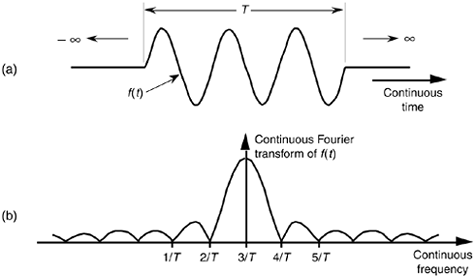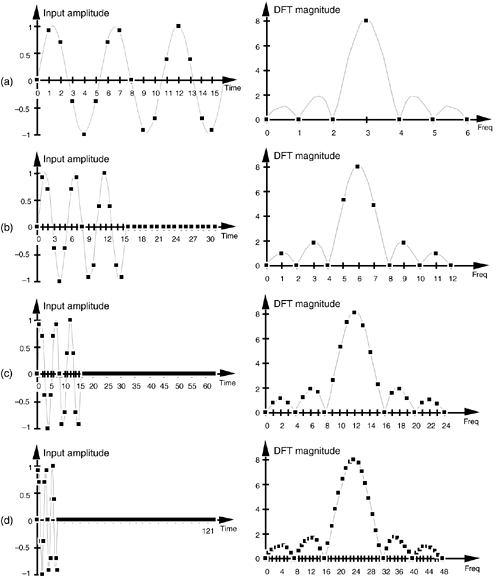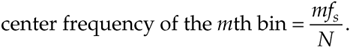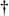# DFT RESOLUTION, ZERO PADDING, AND FREQUENCY-DOMAIN SAMPLING

One popular method used to improve DFT spectral estimation is known as zero padding. This process involves the addition of zero-valued data samples to an original DFT input sequence to increase the total number of input data samples. Investigating this zero padding technique illustrates the DFT's important property of frequency-domain sampling alluded to in the discussion on leakage. When we sample a continuous time-domain function, having a continuous Fourier transform (CFT), and take the DFT of those samples, the DFT results in a frequency-domain sampled approximation of the CFT. The more points in our DFT, the better our DFT output approximates the CFT.

To illustrate this idea, suppose we want to approximate the CFT of the continuous f(t) function in Figure 3-20(a). This f(t) waveform extends to infinity in both directions but is nonzero only over the time interval of T seconds. If the nonzero portion of the time function is a sinewave of three cycles in T seconds, the magnitude of its CFT is shown in Figure 3-20(b). (Because the CFT is taken over an infinitely wide time interval, the CFT has infinitesimally small frequency resolution, resolution so fine-grained that it's continuous.) It's this CFT that we'll approximate with a DFT.

Figure 3-20. Continuous Fourier transform: (a) continuous time-domain f(t) of a truncated sinusoid of frequency 3/T; (b) continuous Fourier transform of f(t).Suppose we want to use a 16-point DFT to approximate the CFT of f(t) in Figure 3-20(a). The 16 discrete samples of f(t), spanning the three periods of f(t)'s sinusoid, are those shown on the left side of Figure 3-21(a). Applying those time samples to a 16-point DFT results in discrete frequency-domain samples, the positive frequency of which are represented by the dots on the right side of Figure 3-21(a). We can see that the DFT output samples Figure 3-20(b)'s CFT. If we append (or zero pad) 16 zeros to the input sequence and take a 32-point DFT, we get the output shown on the right side of Figure 3-21(b), where we've increased our DFT frequency sampling by a factor of two. Our DFT is sampling the input function's CFT more often now. Adding 32 more zeros and taking a 64-point DFT, we get the output shown on the right side of Figure 3-21(c). The 64-point DFT output now begins to show the true shape of the CFT. Adding 64 more zeros and taking a 128-point DFT, we get the output shown on the right side of Figure 3-21(d). The DFT frequency-domain sampling characteristic is obvious now, but notice that the bin index for the center of the main lobe is different for each of the DFT outputs in Figure 3-21.

Figure 3-21. DFT frequency-domain sampling: (a) 16 input data samples and N = 16; (b) 16 input data samples, 16 padded zeros, and N = 32; (c) 16 input data samples, 48 padded zeros, and N = 64; (d) 16 input data samples, 112 padded zeros, and N = 128.Does this mean we have to redefine the DFT's frequency axis when using the zero-padding technique? Not really. If we perform zero padding on L nonzero input samples to get a total of N time samples for an N-point DFT, the zero-padded DFT output bin center frequencies are related to the original fs by our old friend Eq. (3-5), orSo in our Figure 3-21(a) example, we use Eq. (3-32) to show that, although the zero-padded DFT output bin index of the main lobe changes as N increases, the zero-padded DFT output frequency associated with the main lobe remains the same. The following list shows how this works:

Figure No.

Main lobe peak located at m =

L =

N =

Frequency of main lobe peak relative to fs =

Figure 3-21(a)

3

16

16

3fs/16

Figure 3-21(b)

6

16

32

6·fs/32 = 3fs/16

Figure 3-21(c)

12

16

64

12·fs/64 = 3fs/16

Figure 3-21(d)

24

16

128

24·fs/128 = 3fs/16

Do we gain anything by appending more zeros to the input sequence and taking larger DFTs? Not really, because our 128-point DFT is sampling the input's CFT sufficiently now in Figure 3-21(d). Sampling it more often with a larger DFT won't improve our understanding of the input's frequency content. The issue here is that adding zeros to an input sequence will improve our DFT's output resolution, but there's a practical limit on how much we gain by adding more zeros. For our example here, a 128-point DFT shows us the detailed content of the input spectrum. We've hit a law of diminishing returns here. Performing a 256-point or 512-point DFT, in our case, would serve little purpose.[] There's no reason to oversample this particular input sequence's CFT. Of course, there's nothing sacred about stopping at a 128-point DFT. Depending on the number of samples in some arbitrary input sequence and the sample rate, we might, in practice, need to append any number of zeros to get some desired DFT frequency resolution.

[] Notice that the DFT sizes (N) we've discussed are powers of 2 (64, 128, 256, 512). That's because we actually perform DFTs using a special algorithm known as the fast Fourier transform (FFT). As we'll see in Chapter 4, the typical implementation of the FFT requires that N be a power of 2.

There are two final points to be made concerning zero padding. First, the DFT magnitude expressions in Eqs. (3-17) and (3-17') don't apply if zero padding is being used. If we perform zero padding on L nonzero samples of a sinusoid whose frequency is located at a bin center to get a total of N input samples for an N-point DFT, we must replace the N with L in Eqs. (3-17) and (3-17') to predict the DFT's output magnitude for that particular sinewave. Second, in practical situations, if we want to perform both zero padding and windowing on a sequence of input data samples, we must be careful not to apply the window to the entire input including the appended zero-valued samples. The window function must be applied only to the original nonzero time samples, otherwise the padded zeros will zero out and distort part of the window function, leading to erroneous results. (Section 4.5 gives additional practical pointers on performing the DFT using the FFT algorithm to analyze real-world signals.)

To digress slightly, now's a good time to define the term discrete-time Fourier transform (DTFT) that the reader may encounter in the literature. The DTFT is the continuous Fourier transform of an L-point discrete time domain sequence; and some authors use the DTFT to describe many of the digital signal processing concepts we've covered in this chapter. On a computer we can't perform the DTFT because it has an infinitely fine frequency resolution—but we can approximate the DTFT by performing an N-point DFT on an L-point discrete time sequence where N > L. That is, in fact, what we did in Figure 3-21 when we zero-padded the original 16-point time sequence. (When N = L the DTFT approximation is identical to the DFT.)

To make the connection between the DTFT and the DFT, know that the infinite-resolution DTFT magnitude (i.e., continuous Fourier transform magnitude) of the 16 non-zero time samples in Figure 3-21(a) is the shaded sin(x)/x-like spectral function in Figure 3-21. Our DFTs approximate (sample) that function. Increased zero padding of the 16 non-zero time samples merely interpolates our DFT's sampled version of the DTFT function with smaller and smaller frequency-domain sample spacing.

Please keep in mind, however, that zero padding does not improve our ability to resolve, to distinguish between, two closely spaced signals in the frequency domain. (For example, the main lobes of the various spectra in Figure 3-21 do not change in width, if measured in Hz, with increased zero padding.) To improve our true spectral resolution of two signals, we need more non-zero time samples. The rule by which we must live is: to realize Fres Hz spectral resolution, we must collect 1/Fres seconds worth of non-zero time samples for our DFT processing.

We'll discuss applications of time-domain zero padding in Section 13.15, revisit the DTFT in Section 3.17, and frequency-domain zero padding in Section 13.28.

URL http://proquest.safaribooksonline.com/0131089897/ch03lev1sec11AmazonPrev don't be afraid of buying books Next